# sklearn.datasets.load_iris¶

sklearn.datasets.load_iris(return_X_y=False)[source]

Load and return the iris dataset (classification).

The iris dataset is a classic and very easy multi-class classification dataset.

 Classes 3 Samples per class 50 Samples total 150 Dimensionality 4 Features real, positive

Read more in the User Guide.

Parameters: return_X_y : boolean, default=False. If True, returns (data, target) instead of a Bunch object. See below for more information about the data and target object. New in version 0.18. data : Bunch Dictionary-like object, the interesting attributes are: ‘data’, the data to learn, ‘target’, the classification labels, ‘target_names’, the meaning of the labels, ‘feature_names’, the meaning of the features, ‘DESCR’, the full description of the dataset, ‘filename’, the physical location of iris csv dataset (added in version 0.20). (data, target) : tuple if return_X_y is True New in version 0.18.

Notes

Changed in version 0.20: Fixed two wrong data points according to Fisher’s paper. The new version is the same as in R, but not as in the UCI Machine Learning Repository.

Examples

Let’s say you are interested in the samples 10, 25, and 50, and want to know their class name.

>>> from sklearn.datasets import load_iris
>>> data.target[[10, 25, 50]]
array([0, 0, 1])
>>> list(data.target_names)
['setosa', 'versicolor', 'virginica']


## Examples using sklearn.datasets.load_iris¶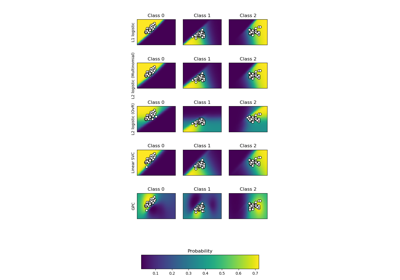Plot classification probability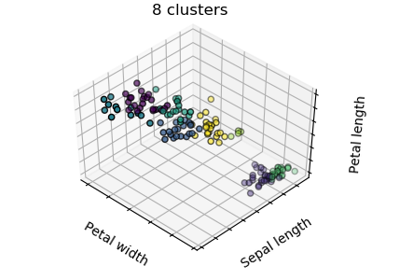K-means ClusteringConcatenating multiple feature extraction methods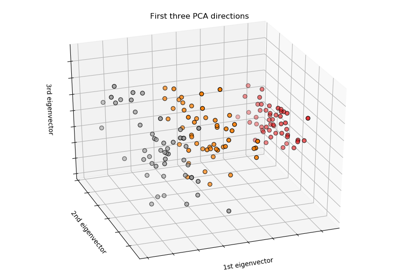The Iris Dataset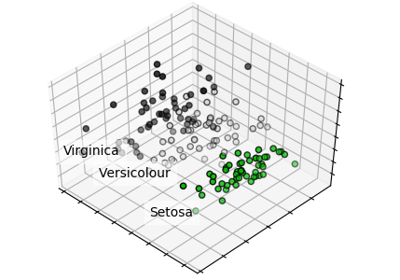PCA example with Iris Data-set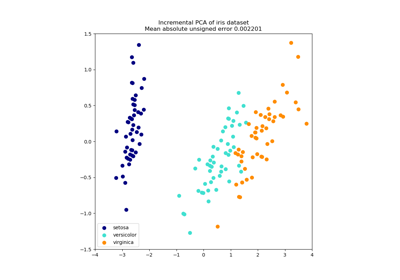Incremental PCA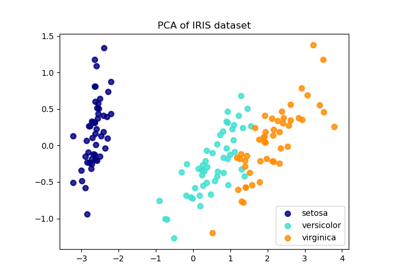Comparison of LDA and PCA 2D projection of Iris dataset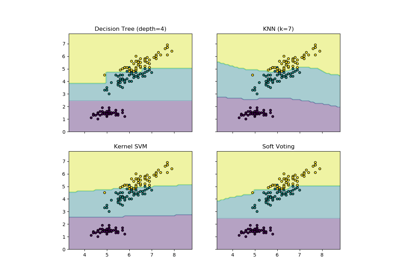Plot the decision boundaries of a VotingClassifier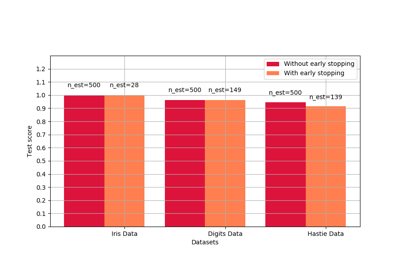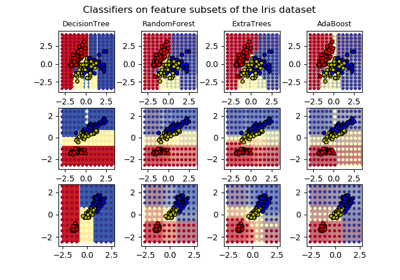Plot the decision surfaces of ensembles of trees on the iris dataset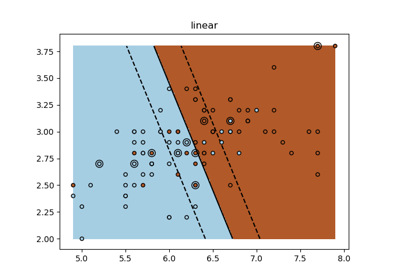SVM Exercise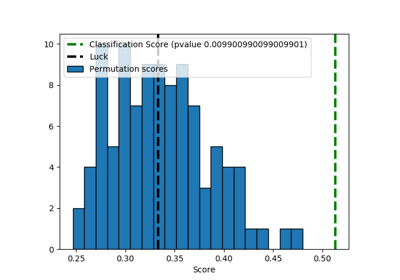Test with permutations the significance of a classification score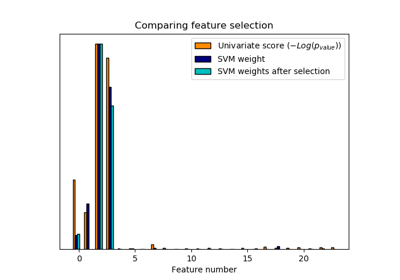Univariate Feature Selection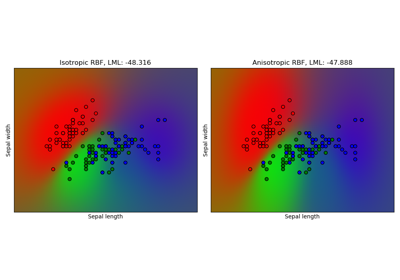Gaussian process classification (GPC) on iris dataset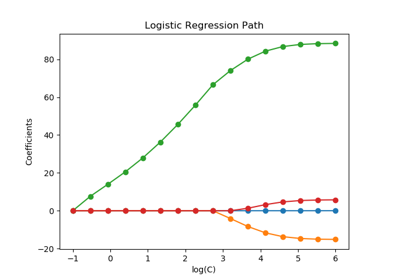Regularization path of L1- Logistic Regression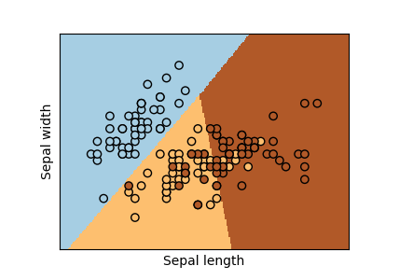Logistic Regression 3-class Classifier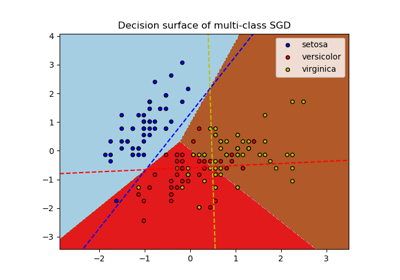Plot multi-class SGD on the iris dataset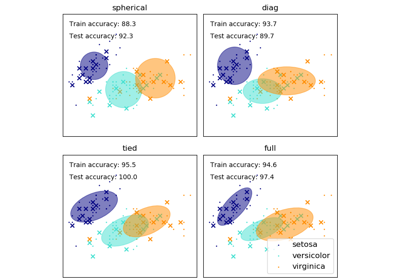GMM covariances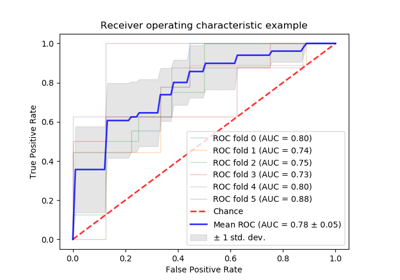Receiver Operating Characteristic (ROC) with cross validation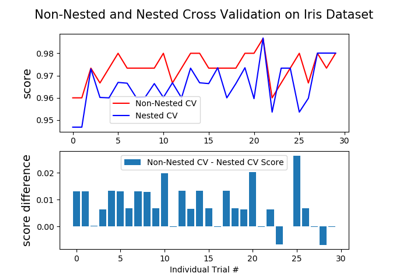Nested versus non-nested cross-validation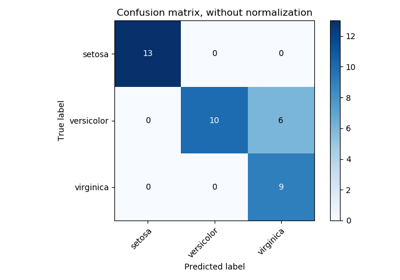Confusion matrix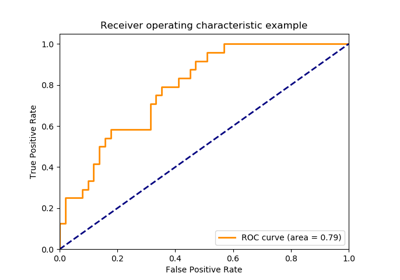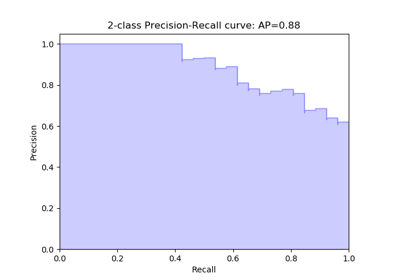Precision-Recall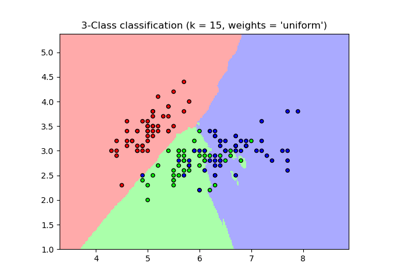Nearest Neighbors Classification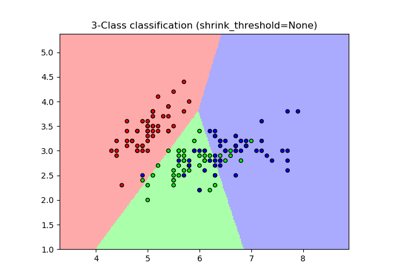Nearest Centroid Classification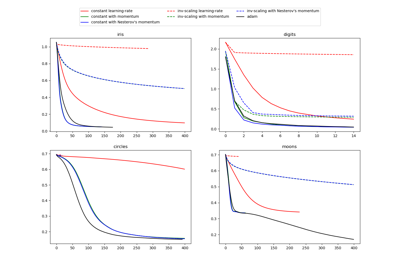Compare Stochastic learning strategies for MLPClassifier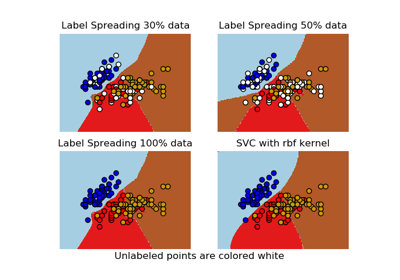Decision boundary of label propagation versus SVM on the Iris dataset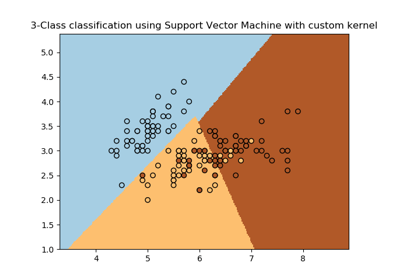SVM with custom kernel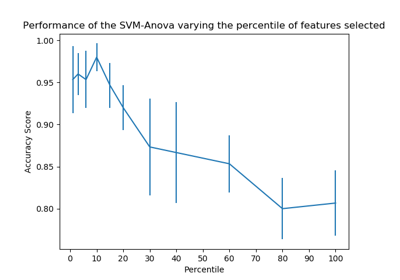SVM-Anova: SVM with univariate feature selection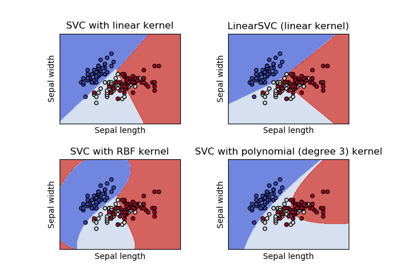Plot different SVM classifiers in the iris dataset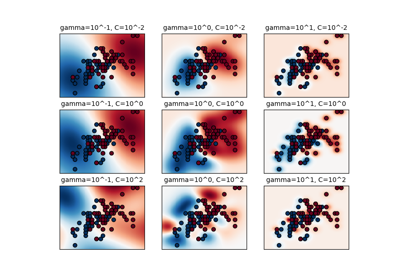RBF SVM parameters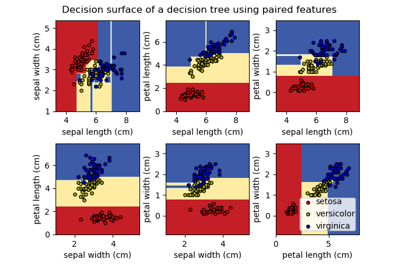Plot the decision surface of a decision tree on the iris datasetUnderstanding the decision tree structure